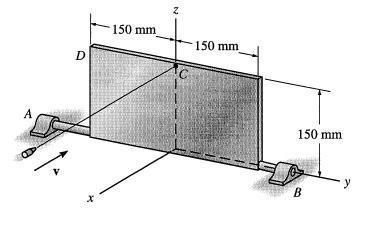Page 1 of 1

### Angular momentum and velocity question, help

Posted: Fri Apr 25, 2008 7:36 am
Hi, this is a question i came across and have been struggling with, some help would be great!

The 15kg plate shown is able to rotate on its axle which is supported on the journal
bearings at A and B. A bullet is fired into the plate providing it with an initial
angular momentum of 9kgm/s. Find the angular velocity of the plate when it has
rotated through 180 degrees from the position shown. (Neglect the mass of the bullet
compared with that of the plate).The main problem i have is im not sure how to get the moment of inertia, im pretty sure i have to work it out about the y axis shown. im not sure if i have to take into account position vectors and such either. anyway any help on where to get started and what equations i need to use would be great.

Thanks

### Re: Angular momentum and velocity question, help

Posted: Fri Apr 25, 2008 8:28 am
Well, as a tip to get started, check out the parallel axis thereom.

Marten

### Re: Angular momentum and velocity question, help

Posted: Fri Apr 25, 2008 9:10 am
That question is a little too engineering oriented for my tastes.

### Re: Angular momentum and velocity question, help

Posted: Fri Apr 25, 2008 10:52 am
is that the linear momentum of the bullet at impact or what? I think the units of angular momentum are multiplied by meters.

### Re: Angular momentum and velocity question, help

Posted: Fri Apr 25, 2008 11:18 am
Assuming that initial L=9, and applying conservation of angular momentum (180 degrees doesnt really matter), I found angular velocity=80.

moment of inertia about side b for a*b rectangular plate is ma^2/3. Yess, it doesnt depend on side b.

### Re: Angular momentum and velocity question, help

Posted: Fri Apr 25, 2008 12:48 pm
180 degrees matters because there is a torque applied by gravity as it rotates.

I used conservation of energy and got 82 s^-1, which is just a slight correction to the answer that didn't take gravity into account.

1/2 * I * wf^2 = 1/2 * I * wi^2 + m*g*h

### Re: Angular momentum and velocity question, help

Posted: Fri Apr 25, 2008 3:30 pm
okay, that makes sense. i see what u mean.
thanks.

### Re: Angular momentum and velocity question, help

Posted: Sun May 04, 2008 4:52 am
wow, that question was apparently a bit tricker than it looked. very astute observation, butsu...

little things like the gravity torque in this problem are the kinds of things we often overlook in GRE problems and get questions wrong because of it... reminds me of a textbook problem that asked us to calculate the final velocity of a positively charged ball that was dropped from a height onto a large negatively charged table... we had just learned the formula for the E field of a plane, and the interesting fact that it's uniform... so all we thought about when solving the problem was the electrostatic energy... and we forgot that gravitational energy was still present.